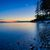# QlikView App Dev

Discussion Board for collaboration related to QlikView App Development.

Announcements
Action-Packed Learning Awaits! QlikWorld 2023. April 17 - 20 in Las Vegas: REGISTER NOW
cancel
Showing results for
Did you mean:Partner - Contributor III

## Total Open items per month

Hi All,

I am trying to build trend for the Total open cases per month. We have the following dimensions.

Case

Case Creation Date

Case Completion Date

In the Chart , we have to show 3 measures:

New Cases:Should be opened in the same month where we are doing Analysis and Case completion date should not be in the same month i.e. beyond that month.

Closed Cases: Cases which has the Completion Date in the same month of Analysis.

Total Cases:

Cumulative number of open cases, starts from 0 at the first point of the selected timeframe (=Total of previous month+New-Closed) -> Total_inMarch=(Total_inFeb + Open_inMarch - Closed_inMarch)

I have attached the sample data .

Any help is appreciated.

Labels (2)

• ### Trend

1 Solution

Accepted SolutionsPartner - Contributor III
Author

Hi All,

Thanks for your response. I managed to find the solution.

We had Case Number with Start and End Date.So first I created one reference date using Start and End Dates called reference date. Reference date is the date for which we are doing analysis.

New Cases for a particular month is counted by in Column 1:

count(distinct(if( (month([Reference Date]) = [Case Created Month]),[Case Number])))

Closed Cases in the Analysis Month is Counted  by in Column 2:

count(distinct(if(month([Reference Date])=month([Case Completion Date]),[Case Number])))

Now we had to find the total Cases Trend per month using  New and closed Cases.

So firstly I calculated the the difference of New and closed cases per month to find the total cases per month remaining using in Column 3:

=Column(1)-Column(2)

after this, Trend of Total cases can be calculated by

=if(Column(1)=0 and Column(2)=0,null(),

if(rowno()<=2,Above(Column(3))-Column(3),Column(3)+Above(Column(4))))

3 RepliesCreator

Hi,

Can you clarify what is Total_inFeb  in this  expression "Total_inMarch=(Total_inFeb + Open_inMarch - Closed_inMarch)". Does it mean total number of cases closed in Feb?MVP
Based on the sample provided, would you be able to provide the expected output as well.Partner - Contributor III
Author

Hi All,

Thanks for your response. I managed to find the solution.

We had Case Number with Start and End Date.So first I created one reference date using Start and End Dates called reference date. Reference date is the date for which we are doing analysis.

New Cases for a particular month is counted by in Column 1:

count(distinct(if( (month([Reference Date]) = [Case Created Month]),[Case Number])))

Closed Cases in the Analysis Month is Counted  by in Column 2:

count(distinct(if(month([Reference Date])=month([Case Completion Date]),[Case Number])))

Now we had to find the total Cases Trend per month using  New and closed Cases.

So firstly I calculated the the difference of New and closed cases per month to find the total cases per month remaining using in Column 3:

=Column(1)-Column(2)

after this, Trend of Total cases can be calculated by

=if(Column(1)=0 and Column(2)=0,null(),

if(rowno()<=2,Above(Column(3))-Column(3),Column(3)+Above(Column(4))))Community Browser